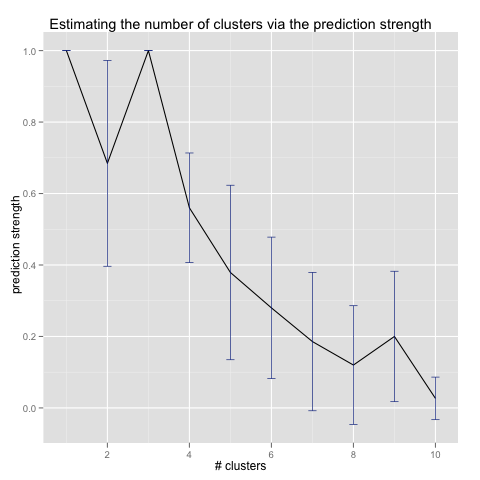Want to share your content on R-bloggers? click here if you have a blog, or here if you don't.

Given a set of numerical datapoints, we often want to know how many clusters the datapoints form. Two practical algorithms for determining the number of clusters are the gap statistic and the prediction strength.

# Gap Statistic

The gap statistic algorithm (from Estimating the number of clusters in a data set via the gap statistic) works as follows. For each i from 1 up to some maximum number of clusters:

1. Run a k-means algorithm on the original dataset to find i clusters, and sum the distance of all points from their cluster mean. Call this sum the dispersion.

2. Generate some number B of reference datasets. A reference dataset can be generated most simply by sampling uniformly from the original dataset’s bounding rectangle; more sophisticated approaches try to sample from the original dataset’s shape more closely, say, by using a rectangle formed from its principal components. Find the mean dispersion of these reference datasets.

3. Define the ith gap by [log(mean dispersion of reference datasets) – log(dispersion of original dataset)].

Once we’ve calculated all the gaps (potentially adding confidence intervals), we select the number of clusters to be the one with the maximum gap.

For example, here I’ve generated three Gaussian clusters:And running the gap statistic algorithm, we see that it correctly detects the number of clusters to be three:(An R implementation of the gap statistic and some more examples can be found at https://github.com/echen/gap-statistic.)

# Prediction Strength

Another cluster-counting algorithm is the prediction strength algorithm (from Cluster validation by prediction strength). To run it, for each i from 1 up to some maximum number of clusters:

1. Divide the dataset into two groups, a training set and a test set.

2. Run a k-means algorithm on each set to find i clusters.

3. For each test cluster, count the proportion of pairs of points in that cluster that would remain in the same cluster, if each were assigned to its closest training cluster mean.

4. The minimum over these proportions is the prediction strength for i clusters.

Once we’ve calculated the prediction strength for each number of clusters, we select the number of clusters to be the maximum i such that the prediction strength for i is greater than some threshold. (The paper suggests 0.8 – 0.9 as a good threshold, and I’ve seen 0.8 work well in practice.)

Here is the prediction strength algorithm run on the same example above:(Again, an R implementation of the prediction strength and some more examples can be found at https://github.com/echen/prediction-strength.)

# Gap Statistic vs. Prediction Strength

In practice, I prefer using the gap statistic algorithm, as it’s easier to code, runs faster, and tends to give slightly better results.